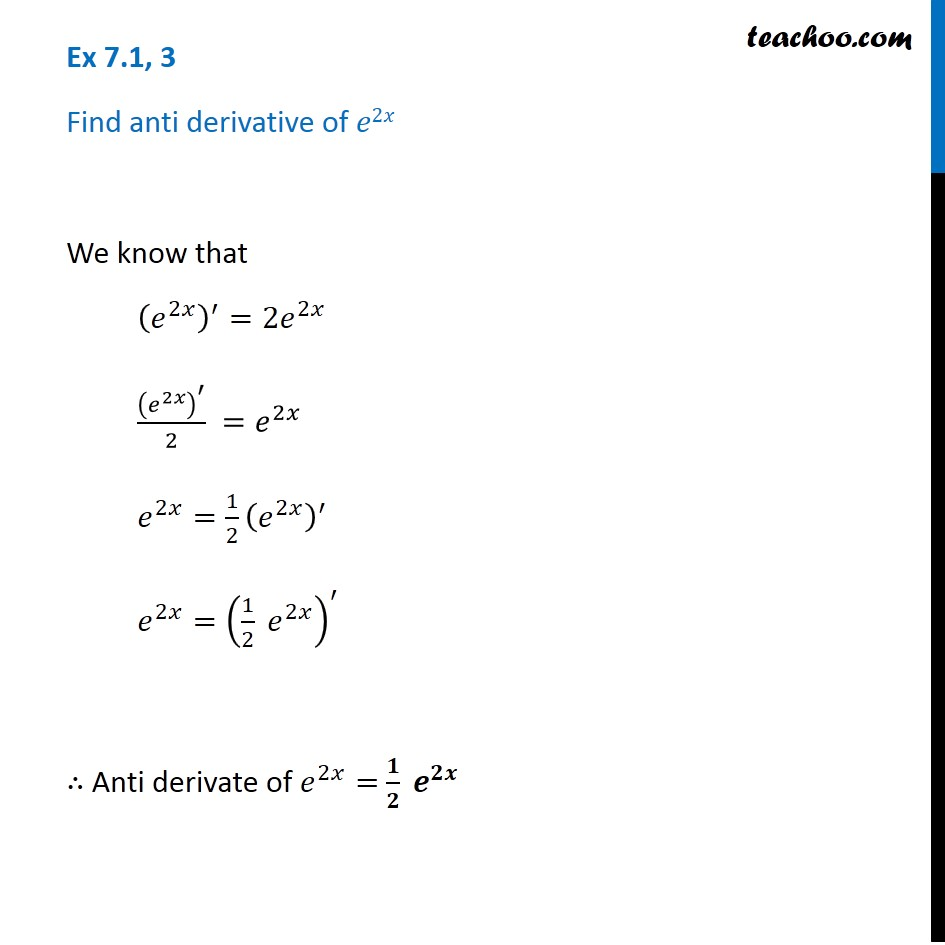Ex 7.1

Chapter 7 Class 12 Integrals
Serial order wiseLearn in your speed, with individual attention - Teachoo Maths 1-on-1 Class

### Transcript

Ex 7.1, 3 Find anti derivative of 𝑒2𝑥 We know that (𝑒^2𝑥 )^′=〖2𝑒〗^2𝑥 (𝑒^2𝑥 )^′/2 =𝑒^2𝑥 𝑒^2𝑥=1/2 (𝑒^2𝑥 )^′ 𝑒^2𝑥=(1/2 𝑒^2𝑥 )^′ ∴ Anti derivate of 𝑒^2𝑥= 𝟏/𝟐 𝒆^𝟐𝒙